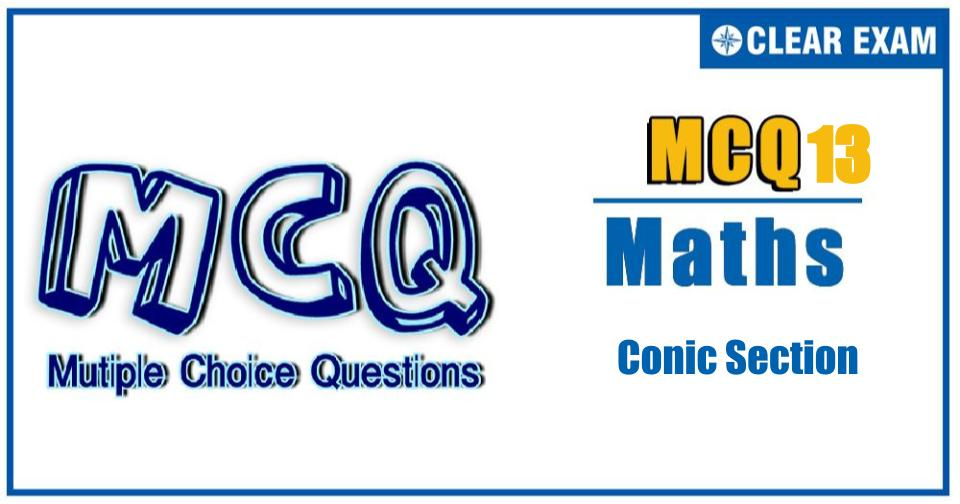[LATEST]\$type=sticky\$show=home\$rm=0\$va=0\$count=4\$va=0

In JEE exams, Conic section is one of the most important topic of Mathematics that comes under Coordinate Geometry, it has total of 15 percent weightage out of which 6% questions are asked in JEE mains and 9% in Advance.

•  (2, 5/2)
•  Data are not sufficient
•  (-2,-5/2)
•  Data are inconsistent
Q2. Minimum radius of circle which is orthogonal with both the circles x2+y2-12x+35=0 and x2+y2+4x+3=0 is
•  4
•  3
•  √15
•  1
Q3. If one of the diameters of the circle x2+y2-2x-6y+6=0 is a chord to the circle with centre (2, 1), then the radius of the circle is
•  √3
•  √2
•  3
•  2

Q4.   Let any double ordinate PNP' of the hyperbola x2/25+y2/16=1 be produced on both sides to meet the asymptotes in Q and Q', then PQ∙P'Q is equal to
•  25
•  16
•  41
•  None of these
Q5. If (0,6)and (0,3) are respectively the vertex and focus of a parabola, then its equation is
•  x2+12y=72
•  x2-12y=72
•  y2-12x=72
•  y2+12x=72
Q6. P is a point on the hyperbola x2/a2 -y2/b2 =1, N is the foot of the perpendicular from P on the transverse axis. The tangent to the hyperbola at P meets the transverse axis at T. If O is the centre of the hyperbola, the OT. ON is equal to
•  e2
•  a2
•  b2
•  b2/a2
Q7. If the locus of middle of point of contact of tangent drawn to the parabola y2=8x and foot of perpendicular drawn from its focus to the tangents is a conic then length of latus rectum of this conic is
•  9/4
•  9
•  18
•  9/2
Q8. Consider the parabola y2=4x. A≡(4,-4) and B≡(9,6) be two fixed points on the parabola. Let ‘C’ be a moving point on the parabola between A and B such that the area of the triangle ABC is maximum, then coordiante of ‘C’
•  (1/4,1)
•  (4, 4)
•  (3,2√3)
•  (3,-2√3)
Q9.If O is the origin and OP,OQ are the tangents from the origin to the circle x2+y2-6x+4y+8=0, then circumcenter of the triangle OPQ is
•  (3,-2)
•  (3/2,-1)
•  (3/4,-1/2)
•  (-3/2,1)
Q10.A straight line moves such that the algebraic sum of the perpendicular drawn to it from two fixed points is equal to 2k. Then , the straight line always touches a fixed circle of radius
•  2k
•  k/2
•  k
•  None of theseWritten by: AUTHORNAME

AUTHORDESCRIPTIONWant to know more

Please fill in the details below:

Latest NEET Articles\$type=three\$c=3\$author=hide\$comment=hide\$rm=hide\$date=hide\$snippet=hide

Name

ltr
item
BEST NEET COACHING CENTER | BEST IIT JEE COACHING INSTITUTE | BEST NEET, IIT JEE COACHING INSTITUTE: Conic Section Quiz-13
Conic Section Quiz-13
https://1.bp.blogspot.com/-IyKDkxV6-8s/YPPY9TV9bNI/AAAAAAAAYPc/NQr4pGei54Y-dXwWrg-JavZvvg7NGh0KgCLcBGAsYHQ/s0/13.jpg
https://1.bp.blogspot.com/-IyKDkxV6-8s/YPPY9TV9bNI/AAAAAAAAYPc/NQr4pGei54Y-dXwWrg-JavZvvg7NGh0KgCLcBGAsYHQ/s72-c/13.jpg
BEST NEET COACHING CENTER | BEST IIT JEE COACHING INSTITUTE | BEST NEET, IIT JEE COACHING INSTITUTE
https://www.cleariitmedical.com/2021/07/conic-section-quiz-13.html
https://www.cleariitmedical.com/
https://www.cleariitmedical.com/
https://www.cleariitmedical.com/2021/07/conic-section-quiz-13.html
true
7783647550433378923
UTF-8